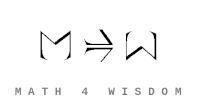文章 发现 ms@ms.lt +370 607 27 665 My work is in the Public Domain for all to share freely. 读物 书 影片 维基百科 Software Upload Make a diagram of concrete mathematical structures that I want to learn about, and related branches of mathematics. Survey combinatorics, especially Stanley's book and Wikipedia, for the various kinds of combinatorial constructions. 学习数学 Priorities: What to learn What are the fundamental math principles? Study the theorems of plane geometry, universal hyperbolic geometry, etc. Organize the most important math theorems and analyze their content in terms of more basic ideas. What are the elementary math concepts? Study how important math concepts relate more basic concepts. Relate basic concepts and more advanced concepts to duality. What is a perspective? Short and long exact sequences. Fiber bundles, vector bundles. Homology and cohomology. Tensors. Sheaves. What is a geometry? SL(2,C) and Mobius transformations. Differences between affine, projective, conformal, symplectic geometry. Classical Lie groups. What is going beyond oneself? Study finite fields and interpret {$F^{1^n}$}. Study points at infinity and how they relate to coordinate systems. What is the foursome? Yoneda lemma. Yates index set theorem. Overview: Math to learn and use Understand the ways of figuring things out binomial theorem: Polytopes, Coxeter groups, homology, Gauss-Bonet theorem, Euler's characteristic, Grassmanian four classical Lie groups, Lie theory, Exceptional Lie groups, Triality four geometries (affine, projective, conformal, symplectic) and how, in logic, they relate level and metalevel. Geometry relation between discrete and continuous projective geometry Symplectic geometry, Lagrangian mechanics, Hamiltonian mechanics the use of variables circle folding, origami and what their operations mean geometrically Numbers: Reals, Complexes, Quaternions, Octonions, Cayley-Dickson construction, Associativity, duality-breaking SL(2,C) and Möbius transformations as the basis for six transformations (reflection, shear, rotation, dilation, squeeze, translation). Visual complex analysis. Network theory, Set theory axioms. Understand how math results unfold math answers A theory of what "equivalence" variously means Foundations: Set theory, Models, Proof theory, Automata theory duality and logic as the relationship between the concious and the unconscious Langlands program, Poincare/Serre duality Brownian motion for modeling the unconscious Symmetric functions for understanding the representation theory of symmetric groups Category theory: Voevodsky, Homotopy type theory, Eduardo Ochs theorems geometry theorems Galois theory Fundamental Theorem of Algebra Spectral sequence in functional analysis. Riemann-Zeta function hypothesis in number theory. P vs NP Qiaochu Yuan's reading list Understand perspectives fiber bundles, vector bundles sheaves in algebraic geometry Grothendieck toposes, Caramello's work Tensor, Triviality, Linear algebra Understand the divisions of everything finite exact sequences Bott periodicity, clock shifts in Clifford algebras, Geometric algebra. higher order homotopy groups for sphere Jacobi identity and universal hyperbolic geometry Yates index theorem and recursive function theory Yoneda lemma Logic Understand representations Grothendieck six operations Understand topologies Mandelbrot set, Catalan for the properties of life Understand the eightfold way Snake lemma, homology and cohomology a unifying perspective on cohomology (or has Lurie already achieved this?) Model how God goes beyond himself Make sense of the field with one element Finite fields, Riemann hypothesis, modeling infinity with characteristic q simplex -1, center and totality, Polytopes entropy as the basis for prayer: Information geometry Completely characterize an area of math such as plane geometry or chess Overviews of Math and Its History Récoltes et Semailles, Part 1, Alexander Grothendieck. Also, translation into Spanish and other works. Notes on the Life and Work of Alexander Grothendieck by Piotr Pragacz A View of Mathematics, Alain Connes Eugenia Cheng. Mathematics, Morally. Thurston. On Proof and Progress. General resources for studying math Math books Are there other nice math books close to the style of Tristan Needham? Websites Kevin Brown collection of expositions of math
Šis puslapis paskutinį kartą keistas November 22, 2021, at 05:40 PM